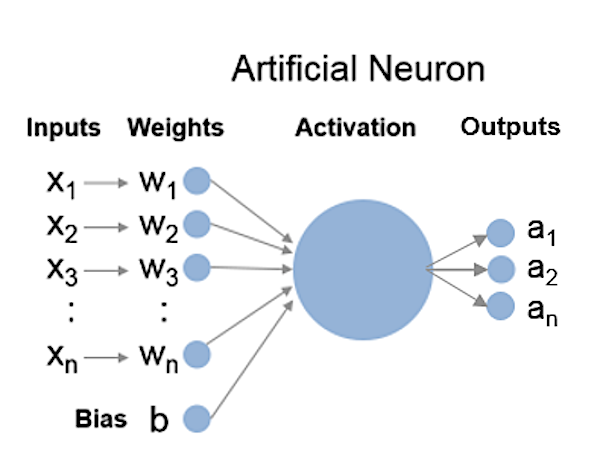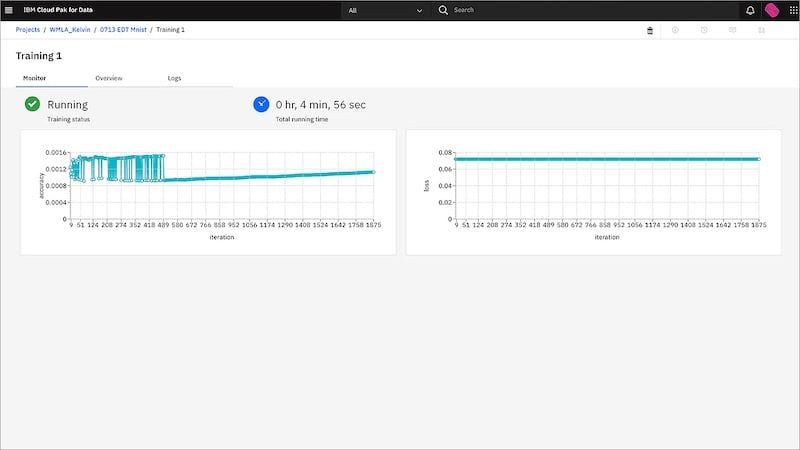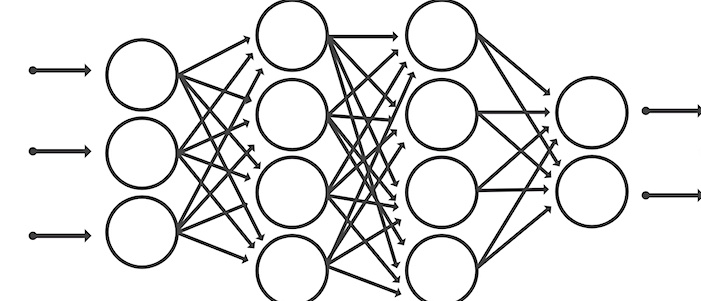Technical Article

# An Overview of Neural Networks

August 18, 2021 by Muhammad Asim Niazi

## What are neural networks, how do you establish basic parameters, and what tools can help?

### What is a Neural Network?

Neural networks are a machine learning technique capable of solving problems without explicitly using human input. They can recognize the inputs, understand user requirements, and produce the desired output. Their processing does not depend on particular programming but on training data fed to them. The training data consists of examples with known answers. The neural network uses these answers to assess and analyze the problem and then processes it.

The way a neural network processes data is similar to the neurons in the brain. The brain neurons learn from the human inputs (such as the senses) and use different processing models from brain memory to solve problems.

Neural networks can be used individually or in combination with other machine learning algorithms. They are successfully being implemented in many practical applications such as speech and image recognition.

### Neural Network Layers

The neurons in the artificial neural network (ANN) are grouped into layers, with each layer responsible for a specific task. Each neuron in a layer has a specific threshold or weight. The corresponding node is activated when the neuron’s value becomes greater than its weight or threshold value. The activated neuron passes data to the next neural network layer.

There are two main layers in a neural network: input and hidden. Another neural network with more than three layers (including input and output) is called a deep learning network.

Following is a brief description of three layers.##### Figure 1. An artificial neuron. Image used courtesy of MathWorks

Input Layer: The neurons in the input layer take the input for processing by the neural networks. There can be more than one input to the neurons in an input layer for a particular application. Each input is assigned a threshold value or weight indicating the significance of that input. Once the input value of a specific node crosses its weight, the node activates and passes the data to the neurons in the next layer (i.e., hidden layer).

Hidden Layer: The neuron in the hidden layer processes the output received from the input layer’s neurons. Neurons In the hidden layer multiply the input by its weight, and then the summation function is activated. The summed variables are then passed to the activation function, which calculates the value.

### Establishing Basic Parameters for the Neural Network

Developing neural networks for various applications depends on the specific requirement in an individual industry. However, generalized steps for neural network development are explained below.

#### Defining Data

There are two types of data required for developing neural networks: training and testing data.

Training Data: This data trains the neural network and defines its workings and characteristics. Training data are sample or example data to make the ANN model learn the deliverables.

Testing Data: This data tests the ANN final model, which results from utilizing training data. The test data is randomly selected from the training data, but does not train the ANN model. Testing data is helpful when selecting a model from the available final models.

#### Assigning Weights and Network Bias

Weights can be defined as the contribution of individual input. It represents the input’s importance level toward the output. Different inputs have different weights. As discussed earlier, each input is multiplied with its corresponding weight and summed. The bias is then added to the sum of the product of all inputs and weights. During training, the weights and biases are randomly assigned.

#### Neural Network Training

During the training stage, the values of weights are adjusted to finetune the output of the neural network to meet user requirements, usually in terms of a maximum error level. The training data is provided to the network, and the output is compared to the target value. Training neural networks starts with unsatisfactory results and gradually moves toward satisfactory results.##### Figure 2. Running a training simulation on Watson Studio. Image used courtesy of IBM

If satisfactory results do not occur, various changes to the structure and parameters of the network can be made. The neural network training is carried out with the help of algorithms; the most common training algorithm is called Stochastic Gradient Descent. The success of training can be measured in various ways relating to error. For example, one method often used with deep learning neural networks is the loss function, which must be lower at the end of the training process.

#### Validation

After neural network training, the network is validated for its performance evaluation with the validation dataset. The validation dataset is used to optimize hyperparameters and control the learning process.

The validation dataset is the sample data collected along with the training dataset, but is not utilized to train the ANN. The validation dataset is selected to qualify the performance of neural networks. In addition to the training model, the output of validation results can amend existing or create a new model.

After all the steps are performed with satisfactory results, a neural network can be put to use.

### Tools for Neural Networks

Sophisticated software tools are available to carry out neural network building, testing analysis, and application. These software tools create an ideal environment for a neural network to build and execute.##### Figure 3. A typical neural network architecture. Image used courtesy of MathWorks

They enable users to execute additional tools on neural networks, such as graphics visualization and code sharing. There are many successful software solutions available, including Matlab and Watson Studio.

#### Watson Studio by IBM

Watson Studio by IBM is an AI and machine learning software that includes neural network functionality as a part of AI applications.

With Watson Studio, engineers can design neural networks with near real-time data. This software allows users to prepare data and build neural networks with open-source code. It also enables debugging different neural network problems and sharing experiments with other engineers and users.

#### MATLAB

MATLAB by Math Works is programming software for analyzing numerical and computing functions. It has a coding environment where engineers and programmers can perform data analysis, visualize graphics, and build algorithms and apps. MATLAB’s working depends on several toolboxes, with each toolbox dedicated to specific applications, such as image processing and digital signal processing (DSP) system toolbox.

MATLAB provides toolboxes for executing neural network functions and applications. These toolboxes are classified under the “AI, Data Science, and Statistics” category. One such toolbox that provides neural network functions is called the “Deep Learning Toolbox.” It provides pre-trained models, apps, and frameworks for designing deep neural networks. Other toolboxes for building neural networks and analyzing functions are the ”Statistics and Machine Learning Toolbox” and “Reinforcement Learning Toolbox.”

Neural networks are complex architectures with layers performing different tasks. These layers can be trained, tested, and validated using tools such as Watson Studio or MATLAB.